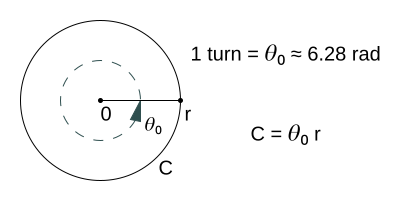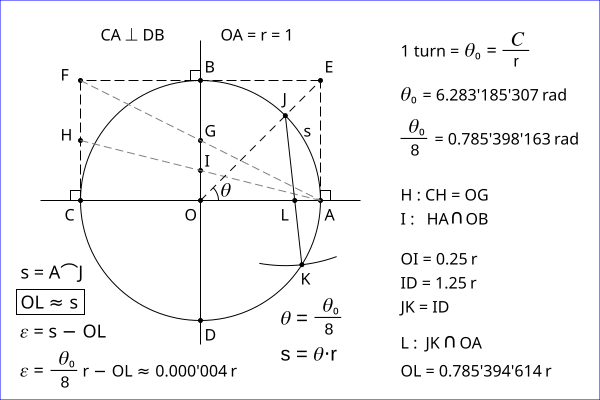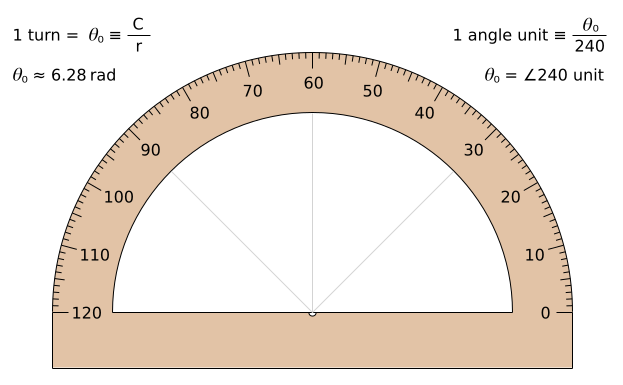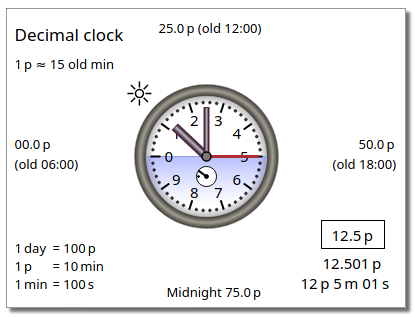# It is a turn

It is a turn to the elegant solutions. I want to describe in this site my opinions and my proposals about the elegant solutions of the different problems.

### •

I agree with Bob Palais that the definition of the well known mathematicel constant pi (𝜋 = C / D) represents a wrong definition of the circle constant. But the number "pi" is not only a confusing and unnatural choice for this constant. There is a deeper theoretical meaning behind the new definition C / r of the circle constant! ...Drawing a circle by a turn and a radius r. The turn (𝜃₀ = C / r) represents a full rotation.

If one turn is always one turn, and a circle is defined by the turn in a distance r, the turn is the circle constant. The circumference of a circle C is given by a turn 𝜃₀ and a radius r: C = 𝜃₀ r
My point of view about the circle constant is in the article
Circle constant is a turn (itisaturn.pdf, updated 2019-04-08).

### •

Maybe you want to see my methods of Squaring the circle.Squaring the circle can be done by the arc length s of the angle 𝜃 = 1 turn / 8.

### •

A simple method for the drawing of the golden ratio and the angle turn/10 is given in my article Golden ratio and the angle turn/10.
Updated 2021-05-05. (See the fig. 4. The fig 4 is renamed 2021-09-01).

### •

Angle unit degree is defined as 1° = 1 turn/360.

Can we have a better definition of the angle unit?Protractor with the angle unit turn/240 . See: A better angle unit
Updated 2022-06-28 (A small text improvement under the Fig. 1).

### •

I have to write about the length units of the pixel grid devices. A pixel of a pixel grid device is always a device pixel. Thus, a pixel is not device independent! The display screens have the different pixel sizes! And the length units are already defined by the metric system. But, the "absolute" length units for the pixel grid devices are redefined by the "device independent pixel". This is the source of many problems. The length unit "device independent pixel" is a problem! A "device independent pixel" represents a constant length value, and it can not represent the device pixels with the different pixel sizes! And there are also many other problems of the "device independent pixel"!The article Pixel grid device units has a solution for the length units of the pixel grid devices.
Updated: 2021-07-05. Text improvement (See: Svg metric test.).

### •

My version of the decimal clock is given by the article Decimal clock Updated: 2022-01-03. A small text improvement of "Decimal time units".Screenshot of the SVG + Javascript version of my decimal clock.